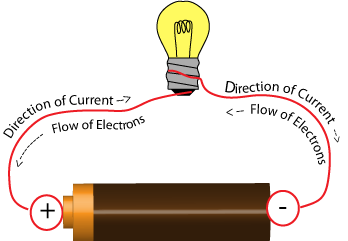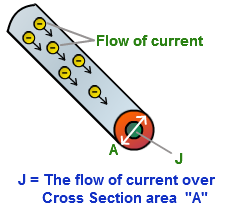Click to Chat

1800-1023-196

+91-120-4616500

CART 0

• 0

MY CART (5)

Use Coupon: CART20 and get 20% off on all online Study Material

ITEM
DETAILS
MRP
DISCOUNT
FINAL PRICE
Total Price: Rs.

There are no items in this cart.
Continue Shopping• Complete Physics Course - Class 11
• OFFERED PRICE: Rs. 2,968
• View Details

Electric Current and Current Density

Table of Content

Electric Current

Types of Electric Currents

Conventional Current

Units of Electric Current

Current Density

Relation between Current Density and Electric Field

Problem (JEE Main)

Related Resources

Electric CurrentWe know that electric potential of a body is defined as the degree of electrification and determines the directions of flow of charge.

Current strength, in a conductor, is defined as the rate of flow of charge across any cross section of the conductor.

If a charge ‘q’ flows across any cross-section in ‘t’ second, current i is given by

i = q/t          …... (1)

Electric current flowing through a conductor is associated with magnitude as well as direction. In spite of this, the electric current is considered to be a scalar quantity. Whenever we have to find the resultant current through a wire we have to take the algebraic sum of all the individual currents. Since this rule is valid only for scalar quantities, we take current to be a scalar quantity.

An electric current is usually thought of as a flow of electrons. When two ends of a battery are connected to each other by means of a metal wire, electrons flow out of one end (electrode or pole) of the battery, through the wire, and into the opposite end of the battery.

An electric current can also be thought of as a flow of positive "holes." A "hole" in this sense is a region of space where an electron might normally be found but does not exist. The absence of the electron's negative charge can be thought of as creating a positively charged hole.In some cases, an electric current can also consist of a flow of positively charge particles known as cations. A cation is simply an atom or group of atoms carrying a positive charge.

Relation (1) holds good if the flow of charge is uniform with time. In case of a non-uniform flow, let ‘dq’ be the small amount of charge flowing across any cross-section of the conductor in a small interval of time ‘dt’, then current ‘i’ is given by

i = dq/dt

Unit of current in S.I. is coulomb/sec or ampere.

Types of Electric Currents

(a) Steady Current: A current is said to be steady if its magnitude is constant and its direction always same.

(b) Variable Current: A variable current, in general, is defined as the current which changes in magnitude with time while its direction may or may not change.

Conventional CurrentElectric current is the flow of electrons from the negative terminal to the positive terminal of a cell. This is because electrons are negatively charged and want to move away from the negative terminal and head towards the positive terminal.

When cells were first invented the theory of electron flow mentioned above was unknown. Rather it was incorrectly assumed that the movement was from the positive to negative terminal. Therefore, circuit diagrams showed the current moving from the positive terminal to the negative terminal. We have unfortunately stuck with this convention to this day and so current flow from positive to negative is called, “conventional current flow” and is used when drawing circuit diagrams. By convention the direction of flow of current is taken to be the direction of flow of positive charge. The current in that sense is called conventional current.

Units of Electric Current

(a) C.G.S. electro-static unit (esu):

The current flowing through a conductor is said to be one esu if a charge of 1 esu flows across any of its cross-section in one second.

1 esu of current = 1 esu of charge/1 second

The esu of current is also called stat-ampere.

(b) C.G.S.electro-magnetic unit (emu):

The current flowing through a conductor is said to be one emu if a charge of 1 emu flows across any of its cross-section in one second.

1 emu of current = 1 emu of charge/1 second

The e.m.u of current is also called abampere.

(c) S.I. unit (ampere):

The current flowing through a conductor is said to be 1 ampere if a charge of 1 coulomb flows across any of its cross-section in one second.

So, 1 ampere = 1 coulomb/ 1 second

Relation between ampere and statampere (esu)

We know that,

1 coulomb = 3109 esu of charge

Thus, 1 ampere = 1 coulomb/1 second = 3109 esu of charge/1 second

1 A = 3109 esu of current or stat-ampere

Relation between ampere and ab-ampere

We know that,

1 coulomb = 1/10 emu of charge

1 ampere = 1 coulomb/1 second

1 A = [(1/10) emu of charge]/[1 second] = (1/10) e.m.u. of current or abampere

Current DensityIn case of a steady current, flowing through a conductor, same current flows through all the cross-sections of the conductor, even though the cross-sections may differ in area. Electric current is a macroscopic entity. We talk of electric current through a conductor and not about electric current at a point. A corresponding microscopic entity, in electricity, is current density.

Current density , at any point inside a conductor, is a vector quantity whose magnitude is equal to the current per unit area through an infinitesimal area at that point, the area being held perpendicular to the direction of flow of charge and its direction is along the direction of flow of positive charge.

Let ‘?I’ be the small amount of current flowing through a small area ‘‘?A’, placed perpendicular to the direction of flow of charge, current density J (magnitude) is given by

J = dI/dA       …... (1)

In vector form,         …... (2)

In case of non-uniform flow, current I through any cross-section is

…... (3)

Here the integral denotes the surface integral over the entire cross-section.

The right hand part of the equation (3) can be regarded as flux of current density over a given area, we can also define electric current as follows.

Electric current is defined as the flux of current density over a given area.

Unit of current density is ‘Am-2’.

Watch this Video for more reference

Relation between Current Density and Electric Field

Consider a conductor of length l and area of cross-section A. Let its two ends be raised to potentials V1 and V2 (V1>V2). As a result of this potential difference, an electric field  is set up in the conductor in a direction parallel to the length of the conductor. Difference of potentials across two ends of the conductor is given by

Let V = V1 – V2

So,

Since direction of  and displacement  (of positive charge) is same

Since E is uniform throughout,

…... (4)

If  = current density vector

Here  and  have same directions.

Thus, I = JA cos 0 = JA

V = IR = I (L/σA)                     (Since, R = L/σA)

Here σ = conductivity of the material of conductor.

Substituting for I, we get,

V = JA(L/σA)

Or, V = JL/σ                   …... (5)

From equations (4) and (5), we get,

EL = JL/σ

Or, J = σE                  …... (6)

Since direction of  and  is same throughout, equation (6) can be written, in vector form, as

Thus electrical conductivity can also be defined as electric current density per unit electric field strength.A current taken to be along the direction of motion of electron is called electronic current.

A current taken to be opposite to the direction of motion of electrons is called conventional current. In physics, we often, deal with conventional currents.

Electricity is a form of energy.

Electricity comes from the conversion of energy sources, such as oil, coal and gas.

Wind, water and solar energy are sustainable sources that can be used to generate electricity.

Electricity is used to power household items but it can also be dangerous.

Electric fields work in a similar way to gravity with an important exception being that while gravity always attracts, electric fields can either attract or repulse.

Electric circuits can contain parts such as switches, transformers, resistors and transformers.

Electric current is measured in amperes (amps).

Electric potential energy is measured in volts.

Two positive charges repel each other, as do two negative charges. Opposite charges on the other hand attract each other.

Charge in motion is referred to as current.

Current can exist in many different physical forms because there are many different physical situations in which charge can flow.

Current density is a characteristic feature of a point inside the conductor and not of the whole conductor.

Current is scalar, while current density is a vector quantity.

The SI unit of current density is Ampere per meter square (Am-2) .

Problem (JEE Main)

The current density in a wire is 10 A/cm2 and the electric field in the wire is 5 V/cm. If ρ is the resistivity of the material, and σ is the conductivity of the material then (in SI units)

(a) ρ = 510-3

(b) ρ = 200

(c) σ = 510-3

(d) σ = 200

Solution:

We know that, E = Jρ

So, ρ = E/J

Substitute 500 V/m for E and 105 A/m2 for J in the equation ρ = E/J, we get,

ρ = E/J = [500 V/m] / [105 A/m2]

Or, σ = 105/500 SI units          (as σ = 1/ρ)

= 200 SI units

Thus from the above observation we conclude that, option (d) is correct.

Problem:

If in the above figure, angle between j, current density vector and A, the area vector of cross section is 60o, current i is 10A and area of given cross section is 2 × 10–6 m2. What is magnitude of current density?

Solution:

We are given the area of cross-section as 60o and the current is 10A.

The area of the given cross-section is 2 × 10–6 m2.

Hence, we know the formula

I = |J| |A| cos q (as J is constant over the cross-section, ∫da = A.

Hence, |J| = 1 x 107 A/m2Question 1

One coulomb of electrical charge is contributed by how many electrons?

?(a) 1.6 × 1019

(b) 1019

?(c) 0.625 × 1019

?(d) 1.6 × 1012

Question 2

5 × 1016 electrons pass across the section of a conductor in 1 minutes and 20 seconds. The current is flowing

?(a) 0.1 mA.

?(b) 1 mA.

?(c) 10 mA.

?(d) 100 mA.

Question 3

By convention, the charge on a glass rod that has been rubbed with silk is called

(a) positive

(b) negative

(c) either positive or negative

(d) none of these

Question 4

What is unit of current density?

(a) ampere/meter

(b) (ampere)2/meter

(c)  ampere/(meter)2

(d) (ampere)(meter)

Question 5

The net charge flowing through a cross section of a conductor in unit time is known as

(a) electric potential

(b) volt

(c) resistance

(d) ampereQ.1
Q.2
Q.3
Q.4
Q.5

c

a

a

c

d

Related Resources

You might like to Resistance and Resistivity.

For getting an idea of the type of questions asked, refer the  Previous Year Question Papers.

To read more, Buy study materials of Current Electricity comprising study notes, revision notes, video lectures, previous year solved questions etc. Also browse for more study materials on Physics here.### Course Features

• 101 Video Lectures
• Revision Notes
• Previous Year Papers
• Mind Map
• Study Planner
• NCERT Solutions
• Discussion Forum
• Test paper with Video Solution